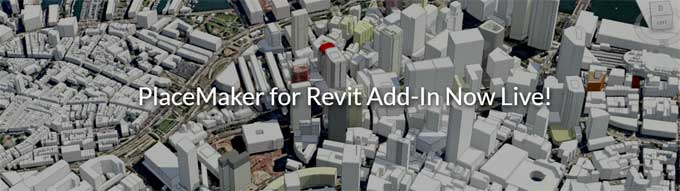# Using a Concrete Rate Analysis Calculator Sheet ProperlyRate Analysis of Concrete: Rate Analysis is called the method of finding the correct and arguably acceptable cost estimate per unit of concreting. There are many factors deciding the price of building per unit area or volume of concrete, and there are many factors involved in it ? from the size of the concrete to what kind of materials are being used to labors to be paid and the instruments to be used. Considering all these matters, determining the resulting cost is called the Rate Analysis of Concrete.

Rate analysis is vital in pre-construction phases since it is a big part of construction cost estimation and cannot be skipped, especially in medium to large projects. Other than concrete, Rate Analysis is also used for brickwork and plastering ? but we will talk about only concrete rate analysis today.

There are seven factors you have to consider to get an accurate rate analysis. These are the following:

Material estimation, Labor estimation, Power/fuel consumption costs, Water supply costs, Equipment rent/purchase cost, Contingencies and safety margin, Overhead and profit estimates.

Now, let us go and see how can we use the concrete rate analysis spreadsheets available in various sites.

A. Specify the area of work: Find the box for the ?area? of concreting that needs to be estimated. Most people do it for 1 cubic meter when doing these calculations by hand, but here you have the ease of inputting any value according to your part of the project.

Let?s assume, it is 10 cubic meters for a supposed project.

B. Select the type of concrete: Since the cost of concrete directly depends upon the type of concrete, you have to select the type of concrete in this part of the project. The type of concrete is generally expressed in terms of the Grade of concrete, which, in turn, is expressed in terms of cement: sand: aggregate ratio.

Let?s say, the ratio is 1:1.5:3.

Note: some spreadsheets automatically calculate aggregate ratio, which should commonly be 2x of sand. If you are using some other kind of concrete with an odd aggregate ratio, you must look for a spreadsheet that allows you to enter specific ratios.

C. Select labor costs: Labors are the actual people who are going to be building your project, and they need to be paid. Figure out how many labors you need and what they need to be paid, and enter that data in the spreadsheet.

There may be a little confusion here, because some rate calculator spreadsheets allow you to enter labor-type specific data, and some allow only to enter the total labor costs. Furthermore, the sheets may allow you to fill in daily data, or total data.

For our example, let?s say we need to pay the following labor charges:

1st Mason: Rs.700/day
2nd Mason: Rs.600/day
Bhisti: Rs.400/day
Coolie: Rs.400/day
Mate: Rs.500/day

D. Material costs: Now that we have specified what type of concrete we are using, we can fill in the price of each material in the sheet. Note that generally cement rate is given as per-bag cost, but sand and aggregate are considered in price per cubic meter. In our present scenario, let us take the most common rates these days:

Cement cost: Rs.320/bag
Sand cost: Rs.1325/cu.m.
Aggregate cost (20mm aggregates, supposing): Rs.1125/cu.m.

E. Find total rate calculation for concrete: We are done entering data! Now is the time to see what result the spreadsheet gives depending upon this data.

In most cases, this should get calculated automatically as you enter the data, in the spreadsheets that use formulas to find the rate. However, some sheets are script based ? or you may be using an application. In that case you may have to click a button or two, depending upon the application.

In any case, if you have entered the example data we have given above, you should be getting the following results (in INR).

Material estimate:

Cement: Rs. 22286
Sand: Rs. 4806
20mm Aggregate: Rs. 8162
Wastage @2%: Rs. 164

Labor charges:

1st Mason: Rs. 350
2nd Mason: Rs. 300
Bhisti: Rs. 1080
Coolie: Rs. 2400
Mate: Rs. 200

Other expenses:

Mechanical mixer rent and running: Rs. 1771
Transportation: Rs. 354
Water: Rs. 398